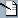LANGREITER.COM plain, simple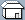START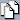INDEXk3-notes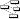CREATED BY chris • LAST EDITED BY chris 5075 days AGO
Note (2007-10-07): Quite a few of the snippets listed here are known to be sub-optimal or interpretative. Use with caution. I finally worked in some improvements Attila Vrabecz sent a long while ago. Thanks again!

I'm keeping old versions for entertainment purposes.

' wonders: www.kx.com/listbox/k/msg04732.html

\ does pointer chasing, as in 0 0 1 0 2\4

& is more generic than I had thought: see &0 1 2 3 5

amend: change items in structure.

monadic amend: a[!3]-: is sugar for @[`a;!3;-;] or .[`a;,!3;-;]
dyadic amend: a[!3]-:x is sugar for @[`a;!3;-;x] or .[`a;,!3;-;x]

a:1 2 3 is sugar for a::1 2 3 (?) / only works outside of lambdas

a[;0] is a[_n;0]. _n selects all.

s[;0] eq s@'0.

{x=y}':s — pairs of equals (1 2 3 3 4 > 0 0 1 0)
=':s / attila

s:();do[1000;s,:{+/1+-2*1000_draw 2}[]] — wanderers
1_1000{+/1+-2*1000_draw 2}\0 / attila

ngrams: (x is alphabet, y is length)
`{(n@*x;#x)}'=n:{y#'(!1-y-#x)_\:x} // doesn't scale{(*x;#x)}'n@=n:{x(!1+-y-#x)+\:!y}`
matrix multiplication (src): (2nd dim held in common)
`{+/''x*/:\:y} / attila: incorrect?(+/*)\: / attila, or just _mul`
slice x into y parts of approximately the same size:
`{n:#x;d:-':c:-1+_1+(!y)*n%y;x@c+!:'d,n-+/d}{n:#x;x@c+!:'d,n-+/d:-':c:-1+_1+(!y)*n%y}{(_(!y)*(#x)%y) _ x}{(y;_%[#x]y)#x} // exact slice`
slice x into parts of size y:
`{x(!y)+/:&0=(!#x)!y} // exact slice{(_%[#x]y;y)#x} // ditto`
moving n-tuples (for each-n-tuple/n-gram fun):
`n:{x(!1+-y-#x)+\:!y}e.g. +/'n[1 2 3;2] is equivalent to +':1 2 3  n["chris";3]("chr" "hri" "ris")`
(fast) moving average ("shift" approach):
`{(y _ x)-(-y)_ x:0.0,+\x%y} // kx.com`
set intersection:
`{?y@&~{(#x)=x?y}[x;]'y}{x@&x _in\:y}{x@&x _lin y} / attila: faster `
set union:
`{?x,y}?, / attila`
multiple occurences:
`{x@c[;0]@&1<#:'c:=x}{x@*:'c@&1<#:'c:=x}`
count occurrences:
`{{(*x),#x}'x@=x}{(*:;#:)@\:/:x@=x}`
split:
`{1_'(&x=y)_ x:y,x}`
e w/ max occurrences in multiset:
`{(?x)@*>#:'=x} // aw`
permutations (algorithm by Roger Hui?):
`{:[1<x;,/(>:'(x,x)#1,x#0)[;0,'1+_f x-1];,!x]}`
(x,x)#1,x#0 is identity matrix, which can be reformulated as id:{a=\:a:!x} (slower!) or id:=\:/2#,!:, leading to:
`{:[1<x;,/(>:'id x)[;0,'1+_f x-1];,!x]}`
permutations (shorter but slower):
`p:{:[x=1;,0;,/a,''(&:'~a=\:a:!x)@\:p x-1]}`
gcd:
`{:[~x%y;y;_f[y;x-y*_ x%y]]}{:[~x;y;_f[y;x!y]]} / attila`
range:
`{*:'x(<x;>x)}{*+x(<x;>x)}`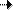www.kx.com/listbox/k/msg05537.html
`10+\\10#1 // binomial coefficients/pascal diamond`
subtract factors (f):
`(0<){x-f@f(*&~>)\x}\`
apply each left (note _n):
`(#:;*:;)@\:1 2 3(#:;*:;).\:,1 2 3(+;-).\:1 2`
hilbert matrix:
`{1%(!x)+\:1+!y}`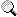SEARCHGET YOUR MOVE ON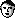ALMOST ALL ABOUT YOU
Nearby in the temporal dimension:
Nobody.
... and 17 of the anonymous kind.
Click on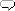for a moderate dose of lcom-talk. This will probably not work in Lynx and other browser exotica.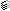k-notes

RECENT EDITS (MORE)films-seenBlood Stoney!keswet towelB StudioPilcrow NewsNastassja Kinski2011-10-06-steve2011-10-06comment-2011-08-04-1

&c.
EDIT